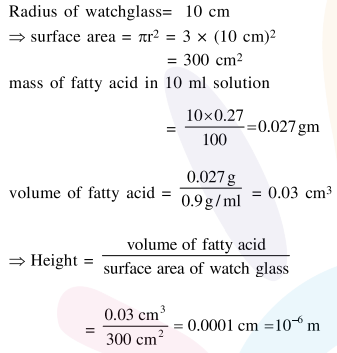Deepak Scored 45->99%ile with Bounce Back Crack Course. You can do it too!

# 0.27g of a long chain fatty acid was dissolved in

Question:

$0.27 \mathrm{~g}$ of a long chain fatty acid was dissolved in $100 \mathrm{~cm}^{3}$ of hexane. $10 \mathrm{~mL}$ of this solution was added dropwise to the surface of water in a round watch glass. Hexane evaporates and a monolayer is formed. The distance from edge to centre of the watch glass is $10 \mathrm{~cm}$. What is the height of the monolayer?

$\left[\right.$ Density of fatty acid $\left.=0.9 \mathrm{~g} \mathrm{~cm}^{-3}, \pi=3\right]$

1. $10^{-8} \mathrm{~m}$

2. $10^{-6} \mathrm{~m}$

3. $10^{-4} \mathrm{~m}$

4. $10^{-2} \mathrm{~m}$

Correct Option: , 2

Solution: